MS 101 Alg & Trig test seven • 15 July 2015 Name:

1. Calculate or solve for x the following expressions and equations.
1. ____________ ${log}_{14}\left(35\right)$
2. ____________ log 316.2278
3. ____________ ex = 12.1825
4. ____________ ln x = 2.5
5. ____________ ${log}_{5}\left(x\right)=2.5$
6. ____________ 6 ln(6x) = 16.2483
7. ____________ ${e}^{\left(\frac{x}{7}\right)}=1.4707$
8. ____________ 2 log(3) − log(x + 144)= −2 log(5)
9. ____________ ${x}^{{\mathrm{log}}_{x}x}=100$
10. ____________ ${log}_{18}\left(12012.562\right)$
2. Sketch a graph of $y=\frac{9}{\left(1+{e}^{-3x}\right)}$ for x = -3 to 3
3.In the Tennis Industry Magazine article Racquet Handle Weighting and Maneuverability Rod Cross notes that, "Inspection of 320 different racquets ... shows that almost every light racquet is head heavy and every heavy racquet is head light." The article goes on to discuss the reasons tennis racquets are designed this way. The following table derives from the article.
Weight (gm)Balance (cm)
24540
26038
28236
30834
34832
1. Add a LOGARITHMIC trend line and equation to the graph. Write the logarithmic equation below:
2. ____________ Based on the logarithmic equation in a, calculate the balance for a weight of 328 gm.
3. ____________ Based on the logarithmic equation in part a, calculate the weight for a balance of 39 cm.
4. _________ Using a 44 foot long rope, Seagal in the center, Tammy walking in a circle with the rope taut, would measure a length of 295 feet. Calculate the value of pi based on this data.
5. _________ Convert 30° to radians expressed as π over a number.
6. _________ Convert 315° to radians expressed as π over a number.
7. _________ Convert $\frac{5\pi }{4}$ radians to degrees.
8. _________ Determine the arc length for a $\frac{\pi }{4}$ arc with a radius of 100.
9. _________ Determine the angle in radians for a radius of 50 and an arc length of 105. The angle can be expressed as a decimal.
10. _________ Calculate: 100 cos(90°)
11. _________ Calculate: 100 sin(90°)
12. _________ Calculate: 100 tan(90°)
13. _________ Calculate: 100 cos(39°)
14. _________ Calculate: 100 sin(39°)
15. _________ Calculate: 100 cos(25°)
16. _________ Calculate: 100 sin(25°)
17. _________ Calculate: 100 cos(13°)
18. _________ Calculate: 100 sin(13°)
19. A heptagon is inscribed in a circle centered at (0,0) with a radius of 100. Determine the SVG coordinates of the vertices of the heptagon A, B, C, D, E, F, G. Write the coordinates in the SVG coordinate system, not the xy algebraic coordinate system.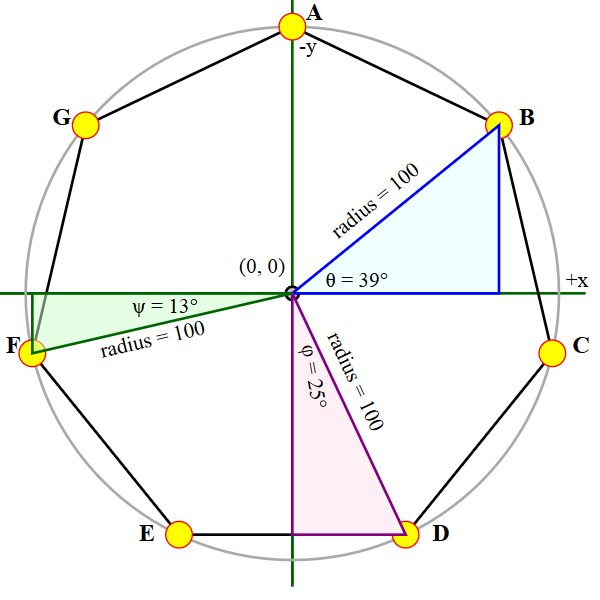1. __________, __________
2. __________, __________
3. __________, __________
4. __________, __________
5. __________, __________
6. __________, __________
7. __________, __________
8. Use SVG and the coordinates above to draw the heptagon inscribed in a circle with a radius of 100. Call me over when you are done so I can see your diagram on your computer.
20. Solve 4 (tan x) − 1 = 0 for x in degrees

21.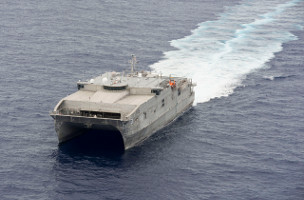The diagram above depicts the sinusoidal wave present in the wake behind the USNS Millinocket JHSV-3 when the ship is underway at sea.
1. _____ About how many wavelengths are present in the wake wave behind the Millinocket?
2. λ = _______________ What is the wavelength λ of the wake wave?
3. a = _______________ What is the amplitude a of the wake wave?
4. b = _______________ Given that $b=\frac{2\pi }{\lambda }$, calculate the value of b for the wake wave.
5. y = ______ sin( _________ x). Given the general form y = a sin(bx) and the results above, write the y = a sin(bx) equation for run A.
22. Calculate the values of θ = ______________ and φ = ______________.
23. A ball is thrown at 260 cm/s perpendicular to the direction of RipStik traveling at 200 cm/s as seen in the diagram. V⃑ground = v⃑ball + w⃑ripstik
1. __________ î + _________ ĵ Write the resultant vector of V⃑ground in unit vector form.
2. __________ Calculate the direction angle θ for V⃑ground in degrees.
3. __________ Calculate the magnitude for V⃑ground.
24. For the vector $\stackrel{⇀}{u}$ = 260 î + 150 ĵ
1. magnitude = __________ Calculate the magnitude.
2. θ = __________ Calculate the direction angle in degrees.
25. A vector with a magnitude of 100 is at a direction angle of 67° to the horizontal. Write the vector in î, ĵ component form.

$\stackrel{⇀}{v}$ = ________ î + ________ ĵ
26. A vector u⃑ with a magnitude of 40 and a direction angle of 30° is added to a vector v⃑ with a magnitude of 50 and a direction angle of 60°. Determine the magnitude and direction angle in degrees for the vector u⃑ + v⃑.
1. magnitude u⃑ + v⃑ = __________ Calculate the magnitude.
2. θ = __________ Calculate the direction angle for u⃑ + v⃑ in degrees.

27.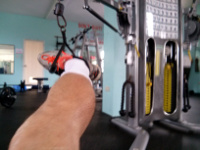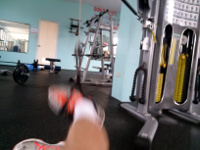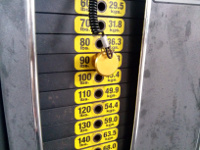__________ Dana is applying a force of 400 Newtons at an angle of 30° to the weight cable for a distance of 0.6 meters. Calculate the energy (work done) using the dot product.
28. __________ Dana is applying a force of 400 Newtons at an angle of 30° to the weight cable. The length of the lever arm is the length of his leg, 0.9 meters. Calculate the torque he is exerting using the cross product.